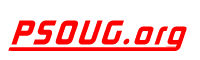Quick Search: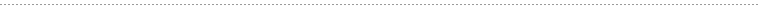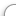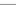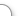CODE Oracle PL/SQL Code Library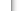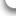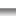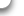JOBS Find Or Post Oracle JobsFORUM Oracle Discussion & ChatThe Oracle VARIANCE Function      [Return To Index] Jump to: Select a Location PSOUG Home Page Oracle Code Library mySQL Code Library PHP Code Library JavaScript Code Library Oracle Terms & Definitions Oracle Error Codes PSOUG Community Blogs Oracle Jobs Board PSOUG Forum Oracle User Group Directory Free Oracle Magazines Online Learning Center PSOUG Presentations Advanced Code Search News and Events Sponsors Page Submit Code Contact UsLooking for the original pages? (formerly called "Morgan's Library") You can find them here.# Term: VARIANCE

Definition:
The Oracle VARIANCE function returns the variance or variability of an expression. In other words, VARIANCE computes how much a set of numbers varies or differs within the set. You can use VARIANCE as an aggregate or analytic function.

The Oracle Database calculates the variance of the expression as follows:
As 0 if the number of rows in expr = 1

• As VAR_SAMP if the number of rows in expr > 1
The Oracle VARIANCE, VAR_POP and VAR_SAMP functions are directly related to their standard deviation counterparts, as standard deviation is simply the square root of the variance. STDDEV and VARIANCE are similar in what they return if there is only one element (both will return a zero).

Example Syntax:

`VARIANCE([DISTINCT | ALL] <value>) OVER (<analytic_clause>)`

Example Usage:

```SELECT last_name, salary,
VARIANCE(salary) OVER (ORDER BY hire_date) AS VARIANCE
FROM employees
WHERE department_id = 30;```